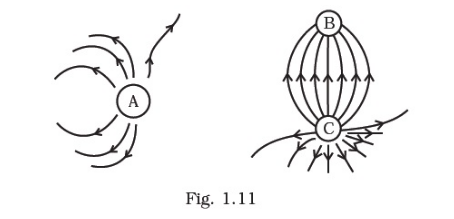# shows the electric field lines around three point charges A,

Question:

shows the electric field lines around three point charges A, B and C.(a) Which charges are positive?

(b) Which charge has the largest magnitude? Why?

(c) In which region or regions of the picture could the electric field be zero? Justify your answer.

(i) near A,

(ii) near B,

(iii) near C,

(iv) nowhere.

Solution:

(a) Charges A and C are positive since lines of force emanate from them.

(b) Charge C has the largest magnitude since maximum numbers of field lines are associated with it.

(c) (1) near A. There is no neutral point between a positive and a negative charge. A neutral point may exist between two like charges. From the figure we see that a neutral point exists between charges A and C. Also between two like charges the neutral point is closer to the charge with smaller magnitude. Thus, electric field is zero near charge A.